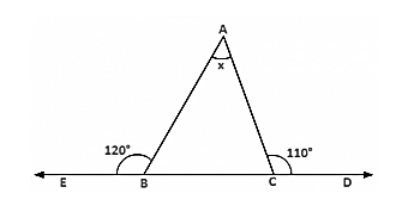# In figure 9.30, the sides BC, CA and AB of a triangle ABC have been produced to D,

Question:

In figure 9.30, the sides BC, CA and AB of a triangle ABC have been produced to D, E and F respectively. If ∠ACD = 105° and ∠EAF = 45°, find all the angles of the triangle ABC.

Solution:∠BAC = ∠EAF = 45° [Vertically opposite angles]

∠ABC = 105° − 45° = 60° [Exterior angle property]

∠ACD = 180° − 105° = 75° [Linear pair]